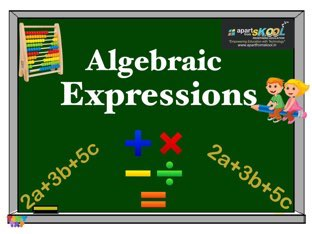# Algebraic Expressions

3,226 Plays
• en-us
• Age: 14+
• 5 years, 8 months ago

An algebraic expression is a mathematical phrase that can contain ordinary numbers, variables (like x or y) and operators (like add, subtract, multiply, and divide).

Play Next:
Smart Play